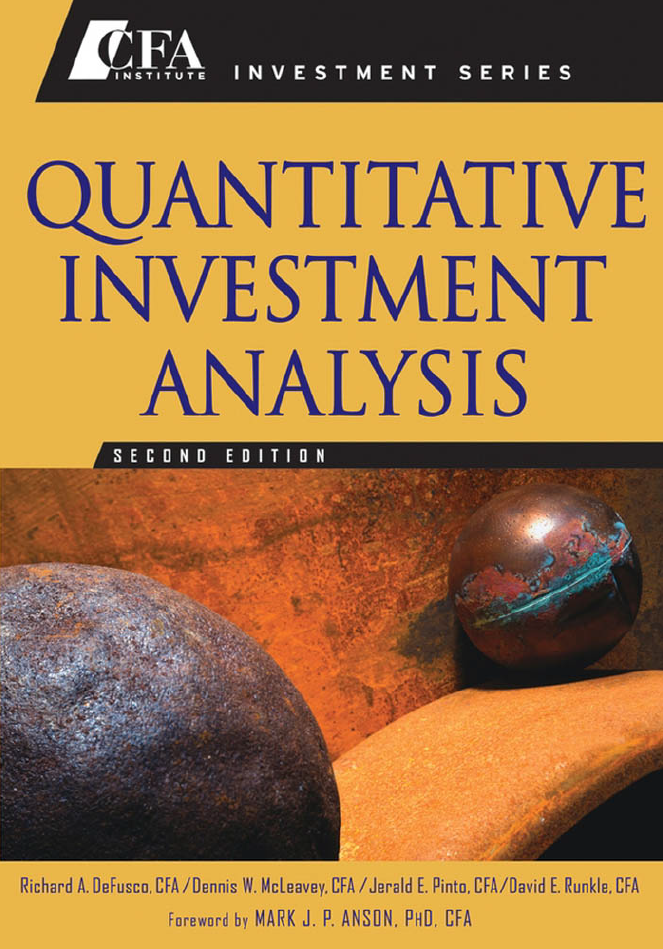23 MAR 2021

# Correlation

Correlation coefficients are one of the most widely used means of determining whether a relationship exists between two variables.

If one of the variables has only a nominal scale of measurement, the correlation coefficient is not usually meaningful. But when the variables have at least ordinal scales of measurement, correlation coefficients provide a useful way of summarizing the relationship between two variables. It should be remembered that the correlation coefficient is a measure of association for a straight line or linear relationship.

Two variables may be related in some nonlinear manner, and the Pearson correlation coefficient does not provide a meaningful manner of describing these nonlinear relationships.

Correlation coefficients are widely used in research work for finance, demographic studies, medical research, and more, allowing the researcher to summarize the relationship between two variables in a single number. A large, or a statistically significant, correlation coefficient can be taken as evidence that the two variables may be causally related. But by itself, a large or a statistically significant correlation cannot prove that two variables are causally related. There is no substitute for determining and understanding how variables are connected, and correlation coefficients cannot indicate this.

In addition to describing relationships, correlation coefficients provide the raw material for many types of multivariate statistical methods. Factor analysis, multivariate regression models, cluster analysis, and other multivariate models can all be built on sets of correlation coefficients.

Formulas and knowledge are taken from:
DeFusco, Richard A. Quantitative Investment Analysis. Wiley, 2007.